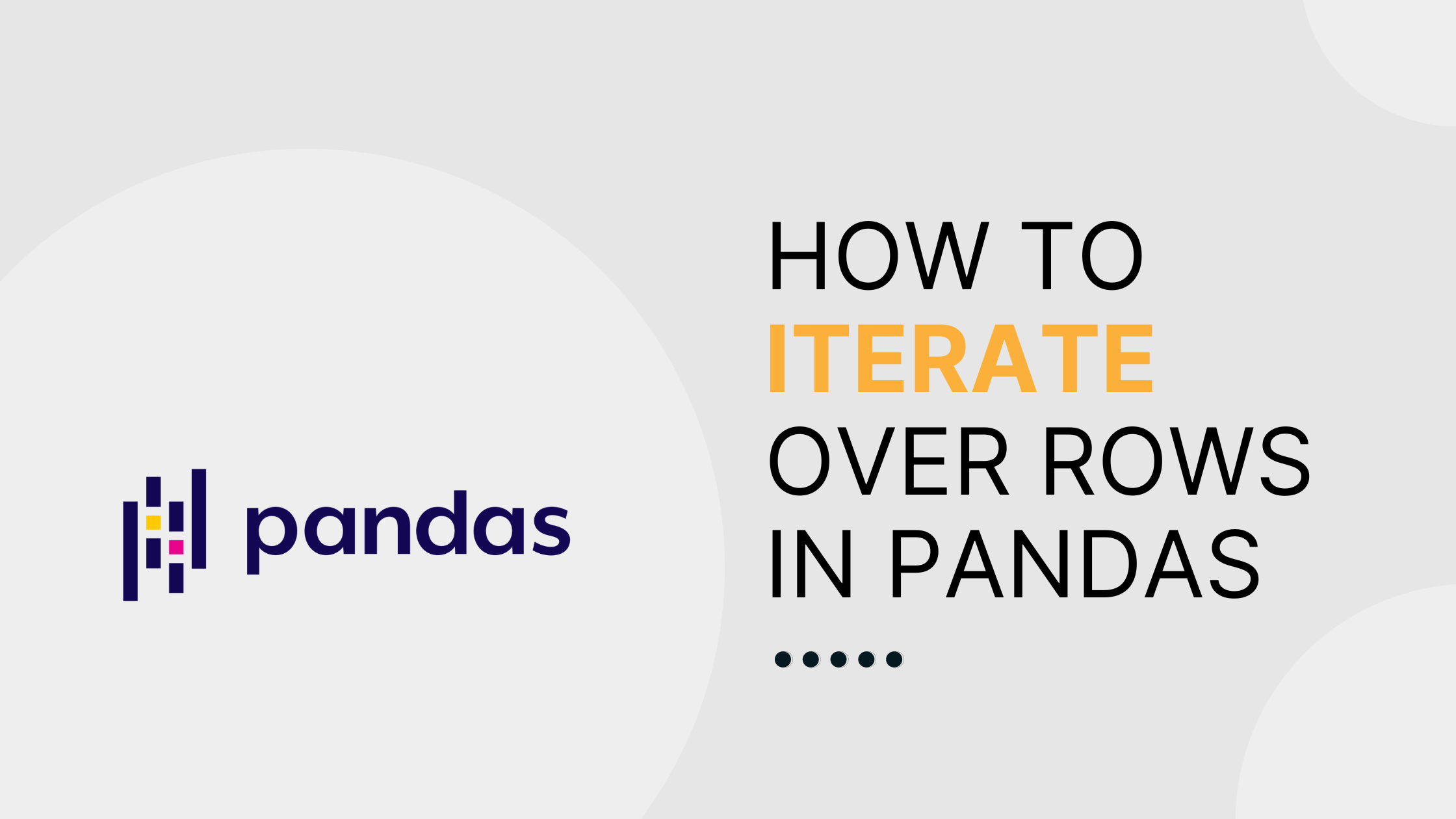# How to iterate over rows in Pandas

Methods for efficiently accessing dataframes by rowWhether you’re a veteran data scientist or trying out the Python package pandas for the first time, chances are good that at some point you’ll need to access elements in your data frame by row. Luckily, Pandas provides the built-in iterators `DataFrame.iterrows `and `DataFrame.itertuples `to help you achieve just that.

`iterrows()` allows you to iterate over rows as (index, Series) pairs, while `itertuples()` allows you to iterate over rows as namedtuples. Here are both in action:

``````import pandas as pd

data = pd.DataFrame({'a': [1, 2, 3, 4, 5], 'b': [10, 20, 30, 40, 50]})

#iterrows
total = []
for index, row in data.iterrows():
total.append(row['a'] + row['b'])

#itertuples
total = []
for row in data.itertuples():
total.append(row.a + row.b)
``````

Note: Because `iterrows()` does not preserve dtypes across the rows, you should never modify something you’re iterating over. If you need to preserve dtypes, use `itertuples()` instead. Additionally, because it uses tuples rather than Panda Series objects, `itertuples()` has a performance advantage over `iterrows()`.

Although the above solutions allow you to iterate over dataframes, iteration is often not the most efficient solution, and in many cases isn’t actually needed at all. While `itertuples()` or `iterrows()` will get the job done on a small dataset (say, a couple thousand rows or less), they are very slow for bigger data. As an alternative, list comprehension can substantially speed up your computation.

``````import pandas as pd

data = pd.DataFrame({'a': [1, 2, 3, 4, 5], 'b': [10, 20, 30, 40, 50]})
total = [a + b for a, b in zip(data['a'], data['b'])]
``````

A still better solution is to vectorize your code. Put simply, vectorization allows you to simultaneously apply a single operation to multiple elements. Vectorized code is not only more efficient than iteration in many use cases, but is also more concise and “Pythonic”, making it easy to read and write. Here are vectorized versions of the code above, using both Pandas and NumPy methods:

``````import pandas as pd

data = pd.DataFrame({'a': [1, 2, 3, 4, 5], 'b': [10, 20, 30, 40, 50]})

#pandas vectorization
total = (data['a'] + data['b']).to_list()

#numpy vectorization
total = (data['a'].to_numpy() + data['b'].to_numpy()).tolist()
``````

To wrap things up, vectorization is much more efficient than iterating over rows in Pandas. If you can’t find a vectorized solution to your problem, you can try using a list comprehension instead. While they are much slower, it’s still worth taking `iterrows()` and `itertuples()` into consideration for small datasets, when dealing with mixed dtypes, or when using `str` functions.